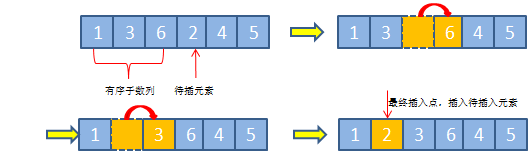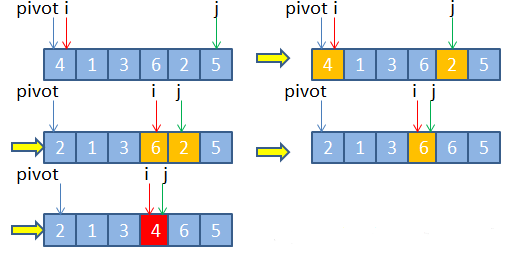# java中插入、分治和快速排序的内容，详细图解

BSO 2020-09-09 08:35:32 java常见问答 9052

java的知识内容庞大而又深厚，这往往需要学习的人花费大量的时间和精力沉浸其中。今天就来为大家介绍一下java中插入排序和分治、快速排序的主要内容，并且通过详细的图片为大家解析。```public class InsertSort
{
public static void sort(int[] arr)
{
if (arr.length >= 2)
{
for (int i = 1; i < arr.length; i++)
{
//挖出一个要用来插入的值,同时位置上留下一个可以存新的值的坑
int x = arr[i];
int j = i - 1;
//在前面有一个或连续多个值比x大的时候,一直循环往前面找,将x插入到这串值前面
while (j >= 0 && arr[j] > x)
{
//当arr[j]比x大的时候,将j向后移一位,正好填到坑中
arr[j + 1] = arr[j];
j--;
}
//将x插入到最前面
arr[j + 1] = x;
}
}
}
}```

1. 选择待排数列的首部第一个元素为基准元素，设置两指针，分别指向数列首尾部位置;

2.假设两指针分别设为i和j;

3.每次遍历的过程如下：

①遍历指针j所指向的元素，直到j指向的元素值小于基准元素时，停止遍历，将其与指针i所指向的元素进行交换，因为当前指针所指位置就是用于插入较基准元素小的元素;②然后再将指针i加一;③接着轮到指针i遍历,直到i所指向的元素值大于基准元素时，停止遍历;④将其与指针j所指向的元素进行交换，之所以可以交换，是因为指针j所指向的元素刚才已经交换到前半部分，所以可以直接选择覆盖。这样就将大于基准元素的元素放于后半部分。⑤依此类推，直到指针i与指针相等或者大于时，停止外部循环。⑥最后直接将基准元素放置于指针i所指向的位置即可，完成分区操作。```public class QuickSort
{
public static void sort(int[] arr, int begin, int end)
{
//先定义两个参数接收排序起始值和结束值
int a = begin;
int b = end;
//先判断a是否大于b
if (a >= b)
{
//没必要排序
return;
}
//基准数,默认设置为第一个值
int x = arr[a];
//循环
while (a < b)
{
//从后往前找,找到一个比基准数x小的值,赋给arr[a]
//如果a和b的逻辑正确--a<b ,并且最后一个值arr[b]>x,就一直往下找,直到找到后面的值大于x
while (a < b && arr[b] >= x)
{
b--;
}
//跳出循环,两种情况,一是a和b的逻辑不对了,a>=b,这时候排序结束.二是在后面找到了比x小的值
if (a < b)
{
//将这时候找到的arr[b]放到最前面arr[a]
arr[a] = arr[b];
//排序的起始位置后移一位
a++;
}
//从前往后找,找到一个比基准数x大的值,放在最后面arr[b]
while (a < b && arr[a] <= x)
{
a++;
}
if (a < b)
{
arr[b] = arr[a];
//排序的终止位置前移一位
b--;
}
}
//跳出循环 a < b的逻辑不成立了,a==b重合了,此时将x赋值回去arr[a]
arr[a] = x;
//调用递归函数,再细分再排序
sort(arr, begin, a - 1);
sort(arr, a + 1, end);
}
}```

java中对象排序的概念是什么?实例代码展示

java Collections类操作集合排序详解

java集合排序该如何实现?